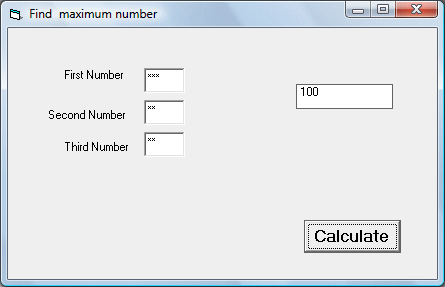# Maximum Number Calculator

This program allows the user to enter three hidden numbers and the program can calculate the maximum number among the three numbers. In order to allow the user to enter the password in hidden mode, you have to set the PasswordChar property to alphanumeric symbols such as * .

You can create a function calMax that consists of three arguments x,y, z .You also need to write a procedure to call this function. This procedure employs the  If Then ElseIf statements and the conditional operators to determine the maximum number. The function Str is used to convert a numeric to string.

### The Interface### The Code

Function calMax(x, y, z As Variant)

If x > y And x > z Then

calMax = Str(x)
ElseIf y > x And y > z Then
calMax = Str(y)
ElseIf z > x And z > y Then

calMax = Str(z)

End If

End Function

Private Sub Command1_Click()
Dim a, b, c
a = Val(Txt_Num1.Text)
b = Val(Txt_Num2.Text)
c = Val(Txt_Num3.Text)

Lbl_Display.Caption = calMax(a, b, c)

End Sub

Private Sub Label5_Click()

End Sub